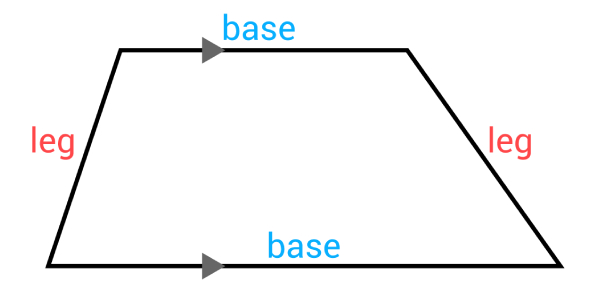# Trapezoids Properties Level Quiz

Approved & Edited by ProProfs Editorial Team
The editorial team at ProProfs Quizzes consists of a select group of subject experts, trivia writers, and quiz masters who have authored over 10,000 quizzes taken by more than 100 million users. This team includes our in-house seasoned quiz moderators and subject matter experts. Our editorial experts, spread across the world, are rigorously trained using our comprehensive guidelines to ensure that you receive the highest quality quizzes.
| By Annacabral
A
Annacabral
Community Contributor
Quizzes Created: 30 | Total Attempts: 18,009
Questions: 10 | Attempts: 859SettingsA trapezoid can be described as a shape that has at least one parallel side. Have you just covered the trapezoid and its properties and are now looking for revision material before the quiz tomorrow? The test below is designed just for you, give it a try. Best of luck it the test!

• 1.

### Which of the following is the correct definition of a trapezoid?

• A.

A quadrilateral with two sets of parallel sides

• B.

A quadrilateral with exactly one set of parallel sides

• C.

A quadrilateral with no parallel sides.

B. A quadrilateral with exactly one set of parallel sides
Explanation
A trapezoid is a quadrilateral with exactly one set of parallel sides. This means that it has two sides that are parallel to each other, while the other two sides are not parallel.

Rate this question:

• 2.

### What is true about an isosceles trapezoid.

• A.

It has exactly one set of parallel sides.

• B.

It has congruent legs.

• C.

It has congruent base angles.

• D.

All of the above

D. All of the above
Explanation
An isosceles trapezoid is a quadrilateral with exactly one set of parallel sides. Additionally, it has congruent legs, which means that the two non-parallel sides are of equal length. Furthermore, it also has congruent base angles, which means that the angles formed by the parallel sides and the legs are equal in measure. Therefore, all of the given statements are true about an isosceles trapezoid.

Rate this question:

• 3.

### Find the measure of angle C in degrees of isosceles trapezoid ABCD

105
Explanation
Since ABCD is an isosceles trapezoid, the base angles A and D are congruent. The sum of the measures of the interior angles of a trapezoid is equal to 360 degrees. Since the base angles are congruent, the sum of angles A, B, C, and D is equal to 360 degrees. Therefore, angle C must be equal to 360 degrees minus the sum of angles A, B, and D. Since angle A is congruent to angle D, the sum of angles A and D is equal to twice the measure of angle A. Therefore, angle C is equal to 360 degrees minus twice the measure of angle A. Since the measure of angle A is 105 degrees, angle C must also be 105 degrees.

Rate this question:

• 4.

### Find the measure of angle A in degrees of isosceles trapezoid ABCD below.

75
Explanation
The measure of angle A in an isosceles trapezoid is equal to the measure of angle D. Since the given answer is 75 degrees, it implies that angle A is also 75 degrees.

Rate this question:

• 5.

25
• 6.

### In isosceles trapezoid ABCD below, AC = 6. What is the measure of diagonal BD?

6
Explanation
In an isosceles trapezoid, the diagonals are congruent. Since AC = 6, the measure of diagonal BD must also be 6.

Rate this question:

• 7.

### In isosceles trapezoid ABCD below, AB = 3x and CD = 12. What does x equal?

4
Explanation
In an isosceles trapezoid, the two non-parallel sides are congruent. Therefore, AB = CD. Given that AB = 3x and CD = 12, we can set up the equation 3x = 12 and solve for x. Dividing both sides of the equation by 3, we find that x = 4.

Rate this question:

• 8.

### Find x in degrees given that Trapezoid ABCD is isosceles.

15
Explanation
Since Trapezoid ABCD is isosceles, the opposite angles are congruent. Therefore, angle A and angle C are congruent. If angle A is given as 15 degrees, then angle C must also be 15 degrees.

Rate this question:

• 9.

### Given that ABCD is an isosceles trapezoid, find the measure of angle A in degrees. First you must find x and then plug it back in.Hint: What is true about angles A and B? (not equal but....)

60
Explanation
Since ABCD is an isosceles trapezoid, the opposite angles A and B are congruent. Therefore, if we find the measure of angle B, we can conclude that angle A is also the same measure. Since the answer is given as 60, we can deduce that angle B is 60 degrees. Hence, angle A is also 60 degrees.

Rate this question:

• 10.

### In the trapezoid shown below, the median EF is drawn in. If BC = 10 and AD = 20, what is the length of median EF?Back to top